# A Complete Guide To Graphing Calculators

May 12, 2021

By  Jacqui

Calculators are an important tool for understanding math. They allow users to compute and complete complex problems in a reduced amount of time. It's recommended to have one if you want to ace your pre and post college exams.

Remember, not all calculators are made equal. Cheaper brands come with flaws that make it hard for students to get used to. You'll want to ensure you fully understand your calculator before taking it to the exam. Getting a high-quality calculator helps you improve your exam scores and are easier to use.

This article is an educational guide made to improve your understanding of graphing calculators. Certain calculators are prohibited by higher education testing grounds. Because of this, our goal is to teach you everything there is to know about graphing calculators.

By the end of the article, you'll have enough information to know which calculator will be the best for you.

## What Is A Graphing Calculator?

Graphing calculators are the newest form of mathematical technology. They are portable handheld devices that are capable of solving simultaneous equations, performing tasks with variables, and plotting graphs.

Since they have larger displays than the average calculator, they can show several lines of calculations and text at the same time. Graphing calculators are programmable, which allows users to create their OWN customized programs, which are typically used for engineering/scientific and education applications.

## Can A Graphing Calculator Be Used As A Scientific Calculator?

There is a clear distinction between graphing and scientific calculators.

Scientific calculators came before graphing calculators. This means that they have smaller screens that show a limited amount of math computations. They are used for chemistry, engineering, and other math-dense fields.

People use scientific calculators because of their ease of use. It doesn't have as many extra feature graphing calculators, making them great for simple math applications. Use scientific calculators when you need a quick answer to a simple math problem.

If you need to handle complex math equations and an accurate graph, you'll want to use a graphing calculator.

Graphing calculators are the modern generation of calculators. Unlike scientific calculators, they can visually show your math computations in an accurate and functional graph. We recommend getting a graphing calculator because they come with extra features tailored to improve your skills in math.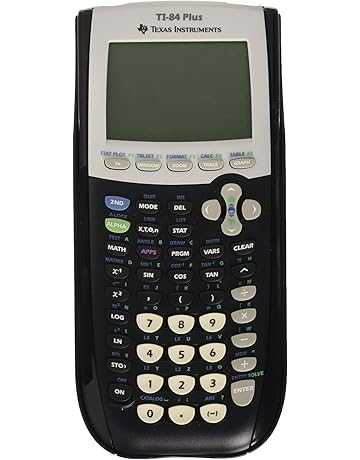To answer the question, you can use a graphing calculator as a scientific calculator. Graphing calculators have superseded scientific calculators because they offer a superset of scientific calculator functionality. This makes it easier for you write down complex scientific notations while being able to showcase your results on a high-quality graph.

## What Is It Used For?

Graphing calculators are great because they can be used for virtually anything that relates to math. It applies to subjects such as Algebra, Geometry, Calculus and in scientific courses such as physics. When getting a graphing calculator, you are getting a versatile tool that can be used for all of your math related needs.

## What Are The Benefits Of Buying A Graphing Calculator?

Before you decide to buy a graphic calculator, you need to know what benefits it can provide to you.

One thing graphing calculators are great at are exposing you to higher level math concepts. For instance, it teaches you how to place your algebraic formulas into a graph for it to be shown. This is great because graphing calculators teach high school students real-world skills that can be used outside of the classroom.

There are a ton of examples we could give here, but let's focus on just one of them. Graphing calculators are great for problems where you need to find the maximum and the minimum. At first, it used to be a challenge for students who had to use calculus techniques to solve these problems.

However, with a graphing calculator, students have found it easier to search and determine the maximum and minimum of a certain problem. Students can focus on how to create and write a function and then use the graphing calculator to search for the maximum and the minimum.

It's a great tool that allows students easy access to higher level skills that are usually seen by colleges.

### Cheaper Than Computers

Admittedly, there are some features a computer has that a graphing calculator cannot replicate. You're more likely to use Microsoft Excel in the real world to do data analysis. That being said, we are not at a point where children need to carry laptops to school because of their expense and size.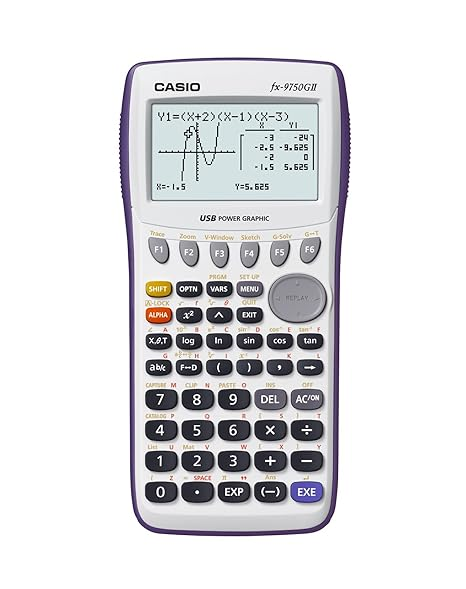As much as popular tech companies such as Google want their products to be the next educational tool, these smartphone devices have a long way from being accepted educational community.

Most schools ban any device with wireless capabilities, and for a good reason. There is a strong desire to cheat with such devices, and they are prohibited from important tests such as the PSAT, ACT and SAT. That's why it's best to just use a graphing calculator for under \$100.

Graphing calculators are cheaper than computers. The average computer costs at least \$600 for it to work properly. Graphing calculators cost at least \$100 on average making it easy on most shoppers budgets.

### Visual & Dynamic

Long gone are the days of a scientific calculator. While they are great for simple math problems, they don't have the ability to showcase results as good as a graphing calculator. Using a graphic calculator gives you the advantage of making your mathematical applications visual and dynamic.

This is a plus because it makes math easier to understand and utilize. For instance, graphing parabolas is a lengthy and tedious process. For some users, it can take up to 30 minutes to graph 6 of them and figure out what each of them is trying to say. Using a graphing calculator helps to solve problems faster, which is important on higher level exams.

Think about it, when creating multiple graphs, how would you want them to appear? Solely using math by hand can take a long time to get the desired result. And on some occasions, you'll often get the wrong answer if you do it that way.

Graphing calculators give you a visual solution that is more accurate and less time consuming than doing the problem manually.

## Graphing Calculator Types

There is a multitude of graphing calculator types that are used for almost any situation. Knowing the difference between them all helps you become a more informed buyer and get the right calculator for you.

### Physical Calculators

Normal calculators are the popular choice amongst students. They offer a wide range of utility and are easy to use during higher level exams. Also, normal calculators are easy to use and allow the student to create complex mathematical solutions.

But, there is one flaw with physical calculators. Some of them don't have any extra software or apps utilized for students.

We suggest using extra online applications to supplement your physical calculator.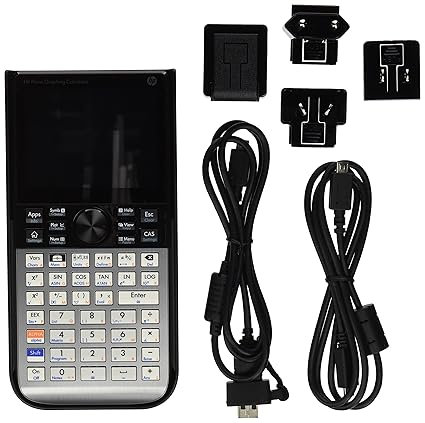They are made to help students learn and understand the complex mechanics behind each math concept.

Physical calculators should be a student's first choice. While online calculators offer some distinct advantages, they are prohibited to use during exam day. Get a physical calculator so that you'll have something to help you during your standardized exams.

### 3D Calculators

Sometimes you need a calculator that displays your graphs in an accurate manner. That's where 3D calculators come in. Students like 3D calculators because they allow them to display their functions in an easy-to-see format.

You'll like 3D graphing calculators if you need something that's easy to manipulate. Most 3D calculators provide students the ability to move and rotate their graphs. Thus, 3D graphing calculators are great if you want the maximum representation of your algebraic graphs.

### Online Graphing Calculator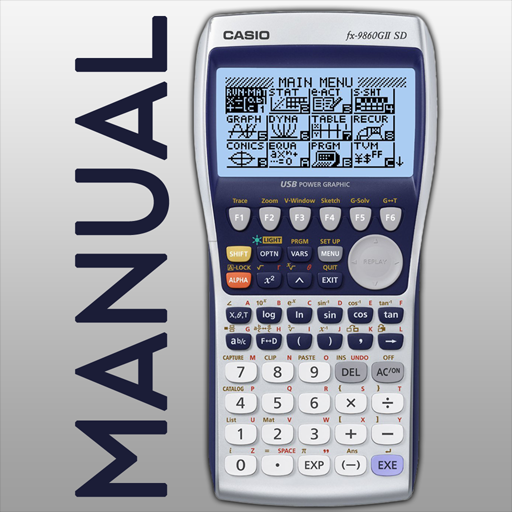When you are at home, try using an online graphing calculator.

They offer the same capabilities and abilities as a physical calculator and are easier to share with other users.

We suggest using Desmos and Mathaway which are reliable software that emulates a standard calculator.

But don't rely too much on online calculators. While they are suitable for creating high-quality graphs, they are not accepted by educational institutions. You'll still need a physical calculator because it's the only tool that higher education exams will accept.

### Online Emulators

Like online graphing calculators, online emulators offer a free alternative to normal devices. The TI-84 emulator is a great example. It gives the user the ability to handle their mathematical computations as if they had a physical copy of the TI-84. Use this emulator if you are planning to get the TI-84, so you'll already know how to use it.

Understand that online emulators and graphing calculators should be used as supplements. They do the same thing as physical calculators, but won't help you during the exam. We stress that getting a physical calculator is important so that you'll be able to have extra help during complicated math tests.

### Apps

Graphing calculators come with extra apps to help further your learning. For instance, some apps such as flashcards allow you to understand and memorize the formulas needed for future exams. See if your calculator comes with additional downloads before buying a graphing calculator.

As we've stated earlier, you don't want to over-rely on apps, online emulators, and online calculators. Most testing institutions prohibit the use of using computer downloaded apps on your device. Having a non-physical or the wrong calculator can result in your score becoming nullified. That's why you need to keep a physical calculator to pass your SAT exams.

## How To Use A Graphing Calculator

Now that you know the different types of calculators, we'll give detailed information on how to use it to your advantage. Knowing how to use your calculator places you a step ahead of other students. We suggest practicing with it an hour a day so that you won't waste exam time trying to use the calculator properly.

Since there are so many graphing calculators out there, we're giving you these details as if you had a TI-84. We've chosen this calculator because it's the most commonly used and offers a lot of benefits for students. It's proven to improve their test scores and GPAs after consistent practice for standardized examinations.

We suggest reading your calculator's instruction manual. This is an important step that most students tend to overlook. It gives you the right knowledge you need to further understand your specific graphing calculator.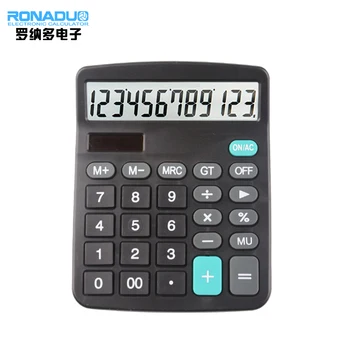### Linear Equations

Solving a group of linear equations can be difficult. Especially when you have more than two equations to be solved. First, you'll want to convert your equations into y-intercept form to graph it correctly.

Remember, the y= mx + b formula? That's what you'll need to convert your equations. If your equation is in standard form (Ax + By = C), then you can substitute the variables so that it fits in the y-intercept form. Just sub in the constants A,B,C and using y = (c-ax)/b.

After that, simply plug the equations into the calculator. When doing this, make sure that the plot points are off unless specialized. The calculator will graph the equation and give you a precise answer to the math problem. Practice linear equations with your calculator to maximize your performance during an exam.

### Linear Inequalities

The next thing you'll want to learn is how to graph linear inequalities. Start by pressing [Y=] located on the top left side of the calculator. Then you need to use the arrow keys to show the function or the sign of the inequality you are trying to define. Press the [ALPHA] and press the key of the desired inequality symbol.

Lastly, you'll want to press the right arrow key and hit enter. This helps you find the definition of the inequality or the function.

You should receive a result like this:

y > 1000 – x

Remember, you can change the inequality sign by following the previous steps. Creating and defining a linear inequality is easier to do on a graphing calculator. Use one if you want to complete linear equality exam questions more accurately and in a reduced amount of time.

### Functions

A good graphing calculator can display mathematical functions fast and accurately. Functions can be graphed or shown on a table. A percentage (15%-20%) of the questions relate to functions, so it's important that you learn how to utilize them on your calculator.

For the sake of this educational guide, we'll show you how to make quadratic, rational, and logarithmic functions with a TI-84 Calculator.

Quadratic functions are defined as an equation. f(x) = ax2+ bx+ c. Each of the numbers in the a, b, and c variables are not equal to zero.

Observe the values for the variables a, b, and c to begin the graph. Make sure each of them is not equal to zero to successfully graph the function.

Next, find the vertex. To locate the vertex, use this formula: x = −b/ 2a. If you want to find the y coordinate, place the value of the x-coordinate in the function.

After setting up your quadratic function, you'll see a parabola displayed on the screen. When graphed, it's shown in a parabola.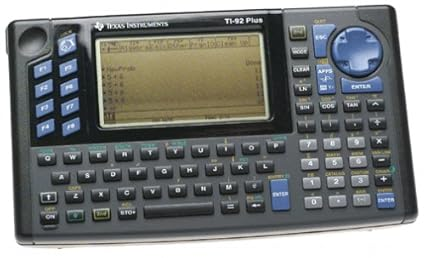Parabolas might vary in steepness or width, but it they still have the basic U-shaped parabola when shown on the graph.

### Using Logarithms

On most exams, you'll see a question involving logarithms. Having a graphing calculator helps you compute and find a solution to these questions.

To do this, go into the [WINDOW] option of your calculator. It should display a number of y1 variables from Y1 to Y7. Here, you'll want to insert the logarithmic function to the right of the equal sign. Press [GRAPH] and you'll see it shown on your calculator's screen.

### Rational Functions

There are only three steps you need to follow to graph rational functions.

• Remove Unwanted Plots
• Create the Function
• Display your graph

Rational functions are the easiest to graph on a calculator. We suggest using a few practice questions to learn how to graph them efficiently.

### Parametric Functions

The last type of functions you'll need to understand is the parametric function. Parametric functions are used to find and express coordinates of the points that make up a geometric object like a surface or a curve. It's important to know how to graph parametric functions so that you'll gain a higher score on your exam.

Here's how you graph a parametric function on your graphing calculator

• Press the [MODE] button on the calculator (located in the second row).
• Press [2ND] [ZOOM] to open up the format menu
• Type X1T = 8sin(T)
• Type Y1T = 4cos(T)

Then you'll see a graph that displays your parametric function. It's a powerful skill to learn on your calculator and will come in handy during exams. Graphing Parametric functions gives you a step ahead in your class and help answer testing questions more accurately.

### Other Uses

Apart from those that are listed above, there are some other uses for a graphic calculator.

#### Simple Arithmetic

Remember, your graphing calculator can be used for simple math arithmetic (Addition, Subtraction, Multiplication, Division). While it's great to create 3D graphs, they are still a great choice for beginners.

For instance, younger students can use graphing calculators to perform basic addition and subtraction problems. Because of this, a graphing calculator is perfect for doing even the simplest level of mathematical applications.

## Manufacturers

Certain graphing calculator manufacturers are accepted by almost all testing sites. You can't go wrong with a calculator from any of them.

Here are a few calculator brands that are accepted by the PSAT, ACT, and the SAT

• Texas Instruments
• Casio
• HP Prime

### Texas Instruments

One of the most recognized calculator brands, Texas Instruments is a highly reliable graphing calculator manufacturer. We suggest getting brands such as the TI-84 because it's easy to use and is 100% accepted on all college testing exams.

### Casio

Casio is the first company to start developing scientific calculators. They have a reputable history, and each of their calculators are designed to maximize your academic performance. Get this calculator if you need an entry-level tool that can handle all sorts of math problems.

### HP Prime

Last on our list is the HP Prime. It's a new calculator developed by one of the most famous computer companies. Students like these calculators because it allows them to perform advanced functions and computations on it. Get a calculator from HP if you are advanced in math and need something to express and create complex math solutions.

## SAT Calculator Policy

You are allowed to use a calculator for the SATs, but it's more than just having a fresh pair of batteries. SAT prohibits the use of laptops, computers, and calclators that have computer downloaded apps located in its data.

Reset your settings back to normal before taking the exam to prevent any issues.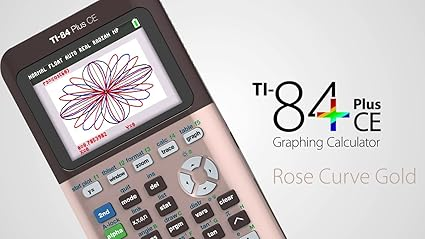## ACT Calculator Policy

The ACT calculator policy is made to ensure that each student has a fair chance when taking their exams. One calculator that's prohibited is the HP Prime, which is a disadvantage for students who only own that calculator. If you have any concerns with your calculator, contact them to confirm if your calculator is permitted.

## NCEES Calculator Policy

This exam is reserved for engineers. To protect the integrity of their exam, NCEES developed a strict policy where only a few set of calculators are permitted.

• Casio: fx-991 and fx-115 models only
• Hewlett-Packard: HP 35 and HP 33 calculators
• Texas Instruments: Any of the TI-36 or TI-30 models

Remember, your calculator must display the name of the model. Getting the wrong model is frustrating and can lead to you failing your exam. Take some time into finding which model from the list above works the best for you.

## Conclusion

To conclude, we believe that a graphing calculator is essential for your academic career. It gives you the ability to construct complex equations and functions with ease. Also, it speeds up the time it takes to answer problems during the exam.

But there is something that still needs to be considered. After knowing the mechanics of a good graphing calculator, you're only halfway done. In the next article, we'll give you a detailed buyer's review to help you find which calculator will work the best for you.

Leave a comment below to let us know what you think.